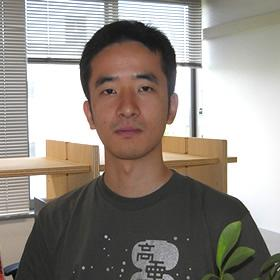Welcome to the Curious World of Science!
Research of Science at Shinshu University.

# Nature and Mathematical Physics

## Current research theme: Mathematical Physics and Quantum Field

--- To high school students ---
(1)Why the materials around us exist stably?
(2)Why quarks cannot be observed?
(3)Why does heat move from higher to lower?
Mathematical physics concerns these subjects. Mathematical physics, which is a part of mathematics, derives various phenomena and physical properties from fundamental mathematical models in a rigorous way.
The problem (1) is clear and no doubt about it in the everyday life. However, the classical mechanics can not be explain the stability of matter, and one needs the quantum mechanics and Pauli exclusion principle. Even if we know the fundamental equation of quantum mechanics, i.e., the many body Schrödinger equation, the proof of stability of matter is a very difficult problem. This problem was mainly solved in the 20th century and the stability of matter was shown under a very wide condition, but some situations are still open.
Problem (2) is the very famous millennium prize problem. The problem (3) looks easy and trivial, but derive the irreversibility of heat and related phenomena is a big problem of mathematical physics.
I'm studying the quantum system of particles interacting with a quantum field, e.g., the system of electron and proton interacting with the electromagnetic field. Let's consider the hydrogen atom which consists of an electron and a proton. Then, the electron is attracted to the proton and moves around the proton. If one does not take into account the electromagnetic interaction, the discrete energy level appear, and there exists a steady states corresponding to the energy levels. In nature, the electron interacting with the electromagnetic field, and the circularly moved electron emits the electromagnetic waves. Then, the problem of stability become the very complex. The accelerated electron creates (and annihilates) infinitely many photons, and some photons are absorbed by the electron and remains may scattered. Moreover, the bare electron is not stable even in the vacuum, and the electron falls down a lower energy state wearing a dress of photon. The electrons we usually observe are this electrons wearing the light. Consequently some properties(e.g. mass) of electron change, and we have to reconsider what was electron in nature. We can not observe this dress of photons, but we can see it through the mathematical formulation of the interaction of electron and electromagnetic field. Even for one electron and light, many mathematical mysteries remain.

## Research Area: Mathematical Physics

The fundamental equation of quantum mechanics is the Schrödinger's equation

$i\frac{\partial}{\partial t}\Psi = (-\Delta+V)\Psi, \quad \int |\Psi|^2dx <\infty$

which was founded in 1926. A few years later, J. von Neumann reveal the mathematical structure of the quantum phenomena, and he formulated the quantum mechanics as the operator theory on the Hilbert space(1932). The quantum mechanics was developed on a infinite dimensional complex Hilbert space which is a vector space equipped an inner product and complete. For example, the vector space

$\mathcal{H} = \Big\{ \Psi:\mathbb{R}^3 \to \mathbb{C} \Big| \int_{\mathbb{R}^3} |\Psi(x)|^2 dx <\infty \Big\}$

with the inner product

$\langle \Psi, \Phi \rangle = \int_{\mathbb{R}^3} \overline{\Psi(x)} \Phi(x) dx$

is a Hilbert space. Any quantum state is written by a nonzero vector in a complex Hilbert space. Here "complex" means that a complex multiple of a vector is also in the space. The complex number plays an essential role in quantum mechanics. Any physical quantity corresponds to an operator on the Hilbert space which maps a vector to another vector. For example, the operator

$H = -\Delta + V(x)$

is the operator corresponds to the energy. and the vector contain complex numbers. Although the Schrödinger equation and state may contain complex numbers, the measured value should be a real number. An operator describing a physical quantity must have a property called the self-adjointness which also allows us the probabilistic interpretation of a state. The conjugate of an operator $T$ having the domain $D(T)$ is defined by

\begin{align*} & D(T^*)=\{\Psi\in\mathcal{H}| |\langle \Psi, T\Phi\rangle |\leq C\|\Phi\| \forall \Psi \in D(T) \}\\ & \langle \Psi, T\Phi\rangle = \langle T^* \Psi, \Phi\rangle, \forall \Psi \in D(T^*), \Phi\in D(T) \end{align*}

The self-adjointness of $T$ is defined by $T=T^*$. Most important theorem for the probabilistic interpretation of self-adjoint operator is the spectrum theorem proved by von Neumann.
Although the mathematical formulation of the quantum mechanics was developed just after the foundation of the Schrödinger's equation, the mathematical analysis began after T. Kato proved the self-adjointness for the Hamiltonian of atomic systems:

\begin{align*} H = \sum_{j=1}^N(-\Delta_j+V(x_j))+\sum_{i < j}\frac{e^2}{|x_i-x_j|}. \end{align*}

Then, the mathematical analysis of quantum mechanics has made great progress, and asymptotic completeness for the many body system with long range potentials including Coulomb force was proved in 1990s.
On the other hand, the mathematical analysis for the quantum field was started around 1950s by A. Wightmann(I don't know the exact year). The initial goal was to construct a quantum field which satisfies the appropriate axioms of quantum field theory. It succeeded in part, but failed for the most important case, i.e., the electromagnetic interactions. But, by abandon relativistic covariance and imposing an appropriate regularization, we can construct the model on a concrete Hilbert space. The first mathematical analysis of such simplified quantum electrodynamics was made by A. Arai in 1980s. Although the methods at beginning were the Bogoliubov transformation, finite volume approximation and probabilistic methods, recently new methods such as an operator theoretical renormalization group methods have been developed.
A beautiful mathematical structure is hidden in the equation describing nature. The fun of mathematical physics is to find it.Itaru SASAKI Mathematics